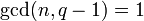# Isomorphism between linear groups when degree power map is bijective

## Statement

### Statement for an arbitrary field

Suppose$K$ is a field and$n$ is a natural number such that the map$x \mapsto x^n$ is a bijection from$K$ to itself. Then, the following are true:

1. The general linear group$GL(n,K)$ is an internal direct product of these two subgroups: the special linear group$SL(n,K)$ and the center$Z(GL(n,K))$, which is the group of scalar matrices and is isomorphic to the multiplicative group$K^*$.
2. The composite of the inclusion of$SL(n,K)$ in$GL(n,K)$ and the quotient map from$GL(n,K)$ to the projective general linear group$PGL(n,K)$ is an isomorphism.
3. In particular, from (2), we get that$SL(n,K) \cong PGL(n,K) \cong PSL(n,K)$.

### Statement for a finite field

Suppose$q$ is a prime power and$n$ is a natural number such that$\operatorname{gcd}(n,q - 1) = 1$. Then the field$\mathbb{F}_q$ of size$q$ satisfies the condition that the map$x \mapsto x^n$ is a bijection, and the following are true:

1. The general linear group$GL(n,q)$ is an internal direct product of these two subgroups: the special linear group$SL(n,q)$ and the center$Z(GL(n,q))$, which is the group of scalar matrices and is isomorphic to the multiplicative group$\mathbb{F}_q^*$, which is a cyclic group of order$q - 1$ (see multiplicative group of a finite field is cyclic).
2. The composite of the inclusion of$SL(n,q)$ in$GL(n,q)$ and the quotient map from$GL(n,q)$ to the projective general linear group$PGL(n,q)$ is an isomorphism.
3. In particular, from (2), we get that$SL(n,q) \cong PGL(n,q) \cong PSL(n,q)$.

## Particular cases$n$ Congruence condition on$q$ for the isomorphisms to hold (basically, need$\operatorname{gcd}(n,q-1) = 1$) Initial examples of$q$
1 all$q$$2,3,4,5,7,\dots$
2$q$ is even$2,4,8,16,\dots$:$SL(2,2) \cong PGL(2,2) \cong PSL(2,2)$ (all are isomorphic to symmetric group:S3)$SL(2,4) \cong PGL(2,4) \cong PSL(2,4)$ (all are isomorphic to alternating group:A5)$SL(2,8) \cong PGL(2,8) \cong PSL(2,8)$ (see projective special linear group:PSL(2,8))
3$q$ is 0 or -1 mod 3$2,3,5,8,9,11,\dots$:$SL(3,2) \cong PGL(3,2) \cong PSL(3,2)$ (see projective special linear group:PSL(3,2)$SL(3,3) \cong PGL(3,3) \cong PSL(3,3)$ (see projective special linear group:PSL(3,3))
4$q$ is even$2,4,8,16,\dots$
5$q$ is not 1 mod 5$2,3,4,5,7,8,9,13,\dots$